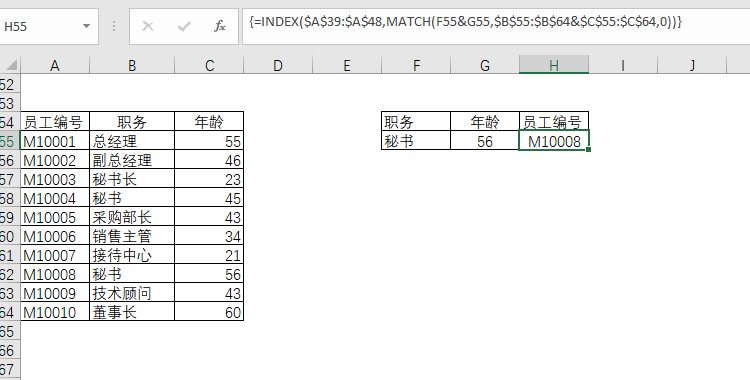## 1 IF类函数

### 1.1 IF函数

IF函数是日常工作中使用Excel时最常用的函数之一，IF函数承载着"如果......那么......否则......"这组关联词的作用。IF函数共有3个参数，每个参数扮演不同的角色，只有参数设置正确，Excel才会明白你的意图。

=IF（判断条件，条件ture返回值，条件false返回值）

1.1.1 单条件判断

E3单元格输入以下公式：

=IF（D3<60,"不及格","及格"）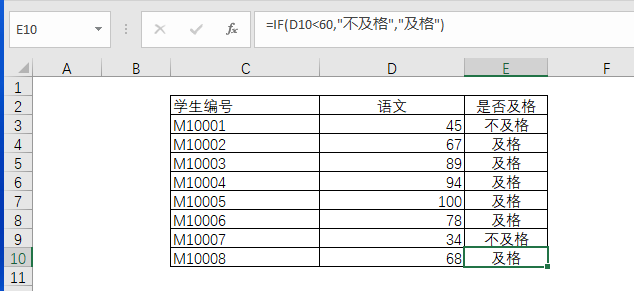1.1.2 多重条件判断

F18单元格输入以下公式：

=IF(D18='物流',IF(E18="车辆管理员","有","无"),"无")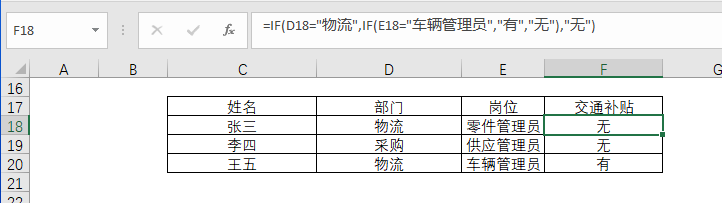### 1.2 SUMIF和SUMIFS函数

SUM是最常用的求和函数，当需要对报表范围中符合指定条件的值求和时，需要用到SUMIF和SUMIFS，它们两者的区别是：

• SUM是直接求和，没有任何条件

• SUMIF是单条件求和，相当于条件筛选一次后求和

• SUMIFS是多条件求和，相当于条件筛选多次后求和

1.2.1 SUM函数

=SUM(F42:F54)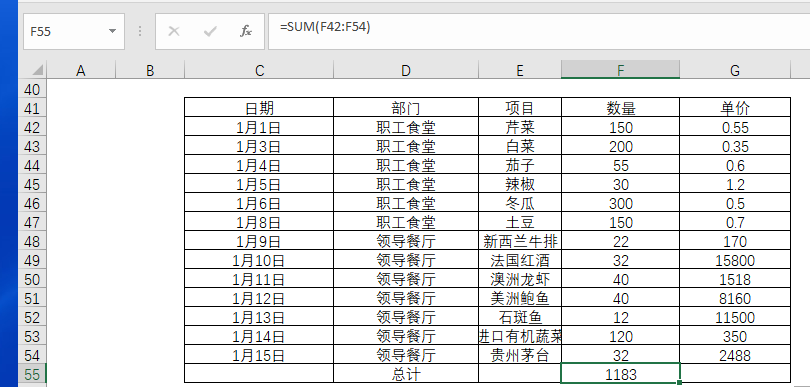1.2.2 SUMIF函数

=SUMIF(条件区域，指定的条件，求和区域)

=SUMIF(42:54,I42,42:54)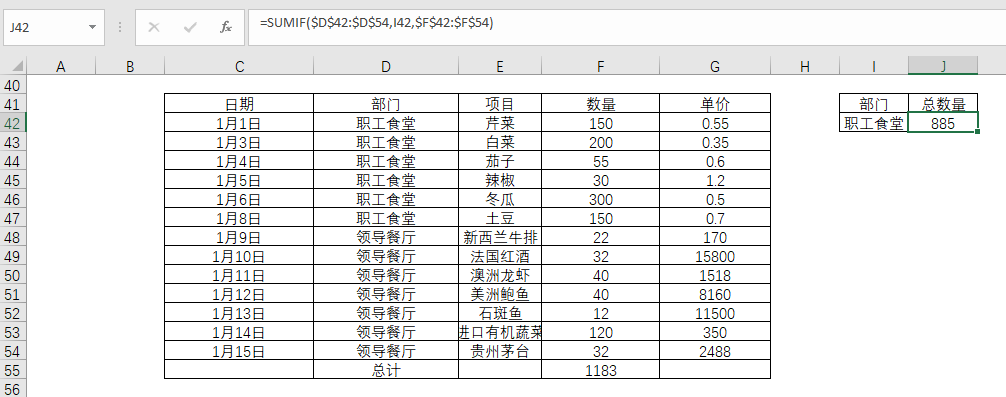1.2.3 SUMIFS函数

SUMIFS函数的作用是多条件求和，这个函数的用法是：

=SUMIFS(求和区域,条件区域1，指定条件1，条件区域2，指定的条件2,......)

=SUMIFS(61:73,61:73,61,61:73,61)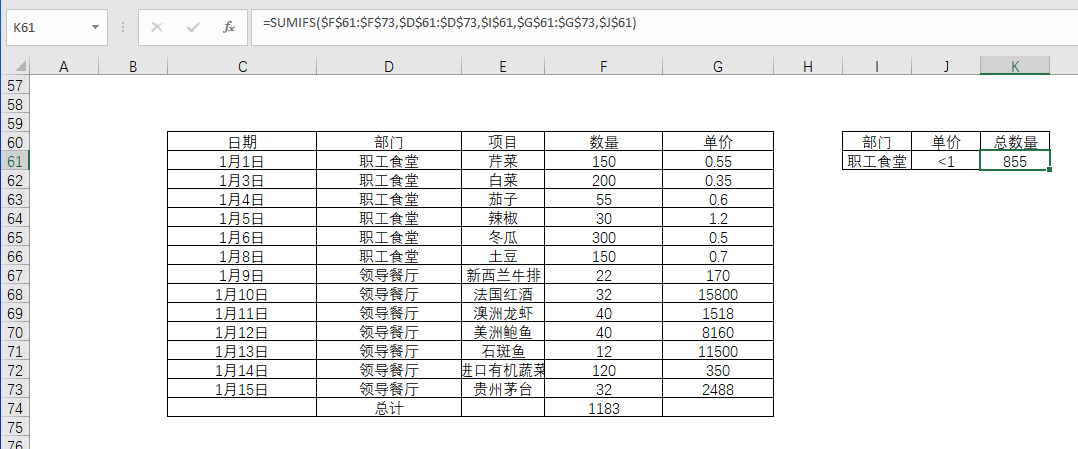### 1.3 IFERROR函数

=IFERROR(VLOOKUP(......),0)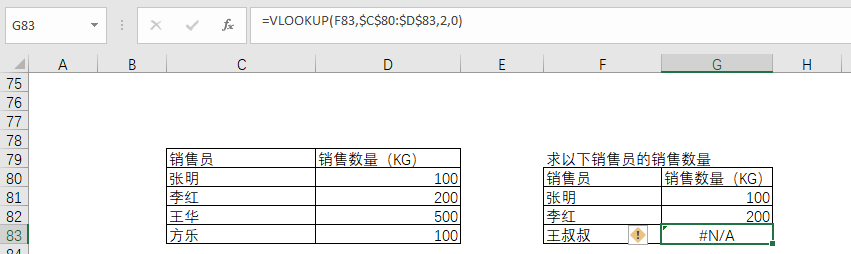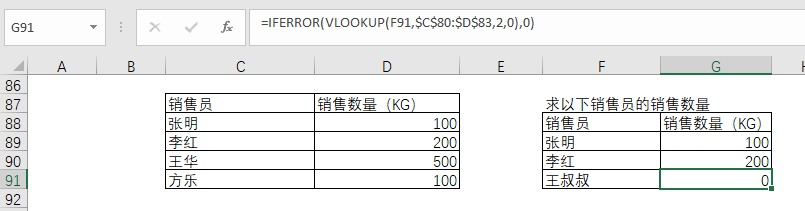## 2 VLOOKUP函数

VLOOKUP函数是Excel中的一个纵向查找函数，它与LOOKUP函数和HLOOKUP函数属于一类函数，在工作中都有广泛应用。比如，当有多张表时，如何将一个excel表格的数据匹配到另外一个表中？这时候就需要使用VLOOKUP函数。

=VLOOKUP(要找谁，在哪儿找，返回第几列的内容，精确找还是近似找)

• 第一个参数是要查询的值。

• 第二个参数是需要查询的单元格区域，这个区域中的首列必须是要包含查询值，否则公式将会返回错误值。如果查询区域中包含多个符合条件的查询，VLOOKUP函数只能返回第一个查找到的结果。

• 第三个参数用户指定返回查询区域中的第几列的值。

• 第四个参数是决定函数的查找方式，如果为0，则是精确匹配方式。如果为1，则是近似匹配方式。

### 2.1 常规查询

=VLOOKUP(H96,96:105,3,0)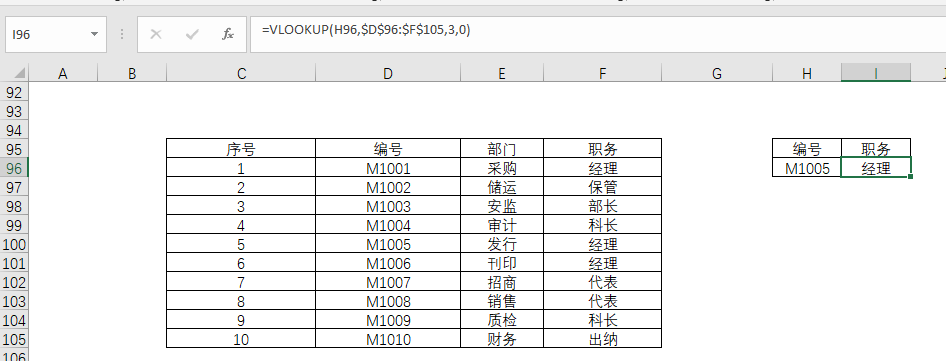### 2.2 带通配符查询

=VLOOKUP(F111,111:114,2,0)

=VLOOKUP(""&F111&"",111:114,2,0)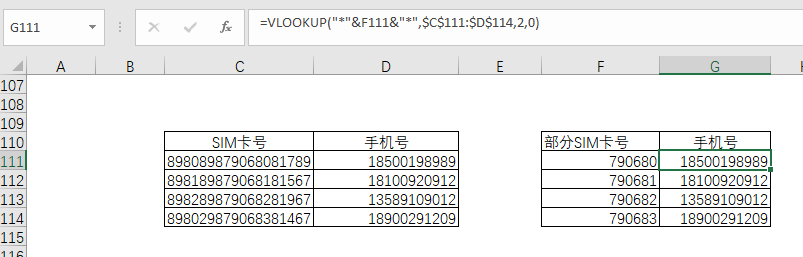### 2.3 近似查询

0-60分，为不合格；60-80分，为合格；80-90分，为良好；90分以上，为优秀。

=VLOOKUP(E120,121:124,2,1)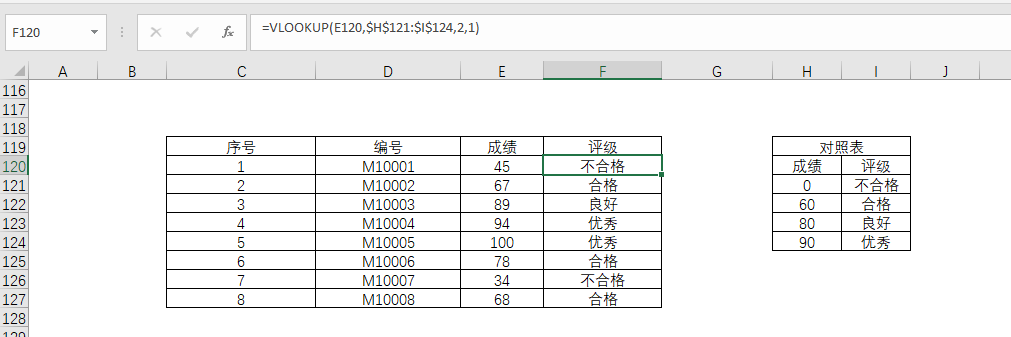### 2.4 逆向查询

=VLOOKUP(H132,CHOOSE({1,2},E132:E141,D132:D141),2,0)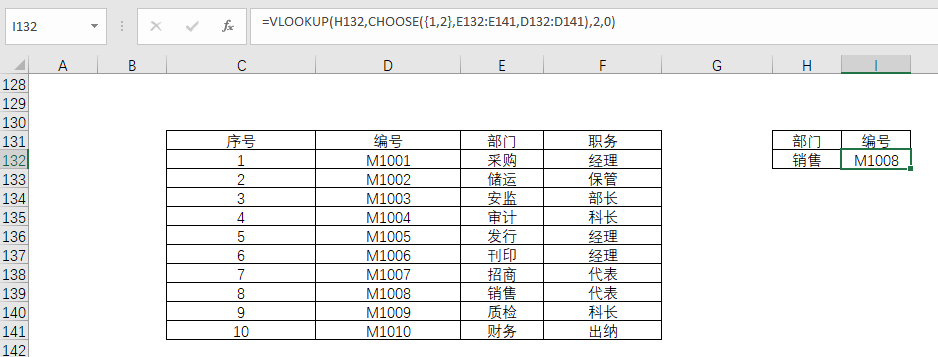## 3 SUMPRODUCT函数

SUMPRODUCT函数是用于在给定的几组数组中，将数组间对应的元素相乘，并返回乘积之和。

=SUMPRODUCT(数组1：数组2：数组3)

=SUMPRODUCT(数组1*数组2)

### 3.1 常规乘积求和

=SUMPRODUCT(C5:C8,D5:D8)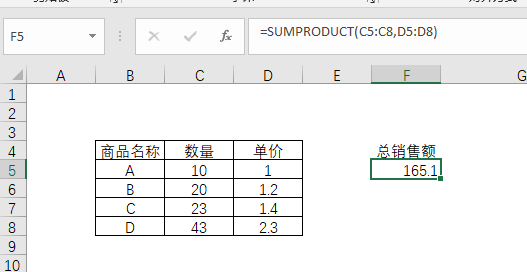### 3.2 多条件计数

25岁及以下女性的人数：

=SUMPRODUCT((16:22<=25)*(16:22="女"))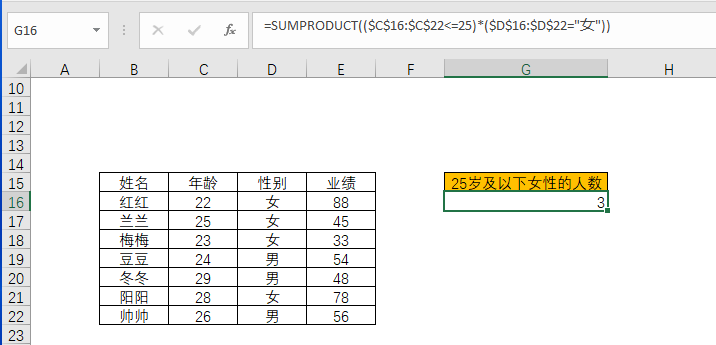### 3.3 多条件求和

25岁及以下女性的业绩：

=SUMPRODUCT((16:22<=25)*(16:22="女"),29:35)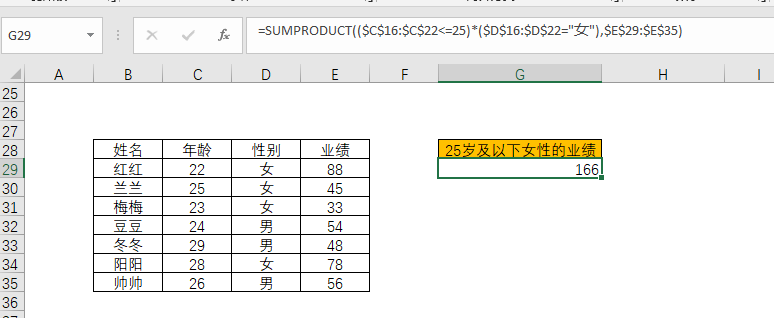### 3.4 二维区域求和

=SUMPRODUCT((42:46="销售1部")*42:46)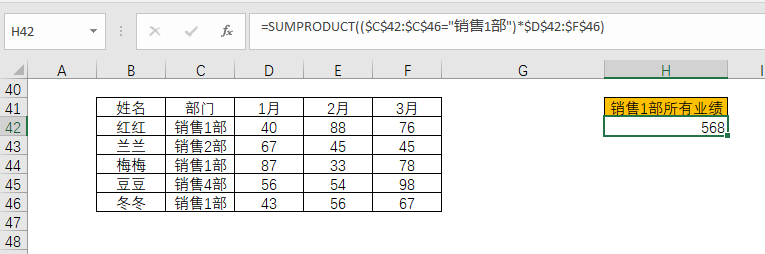### 3.5 二维区域多条件求和

=SUMPRODUCT((42:46="销售1部")*(53:53="3月"),54:58)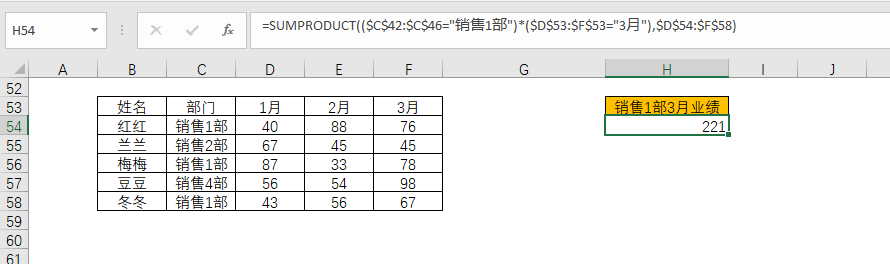## 4 MATCH函数组合

MACTH函数是EXCEL中使用较为广泛的一个函数，MATCH函数的功能就是在指定区域内搜索特定内容，然后返回这个内容在指定区域里面的相对位置。通俗的来讲，就是返回指定值在数值的位置，如果在数组中没有找到该值则返回#N/A。

=MATCH(查找的内容，查找的区域，匹配类型)

• 1或省略，查找小于或等于指定内容的最大值，而且指定区域必须按升序排序

• 0，查找等于指定内容的第一个数值

• -1，查找大于或等于指定内容的最小值，而且指定区域必须按降序排序

=MATCH(100,148:151,1)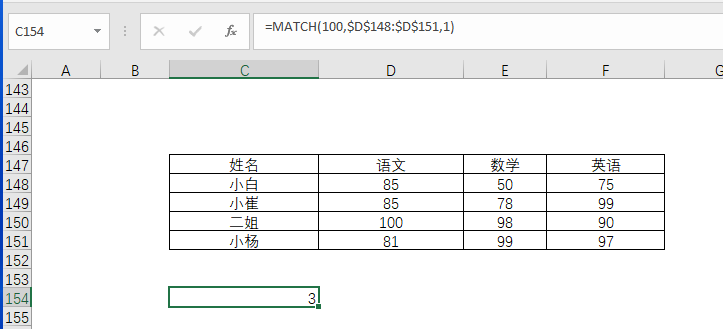=MATCH(80,159:162,1)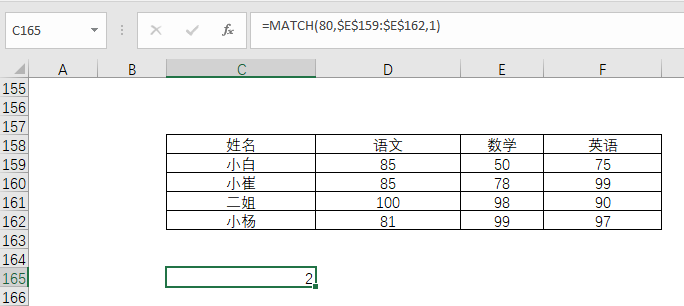=MATCH(90,170:173,-1)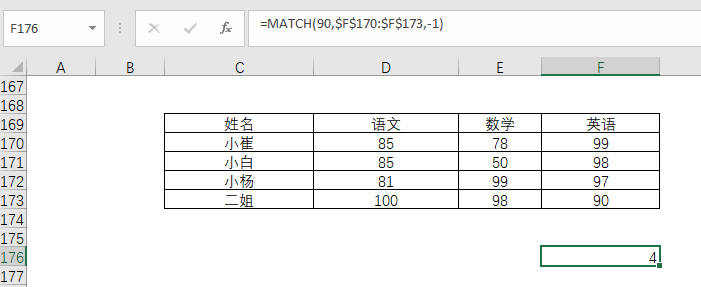### 4.1 MATCH与OFFSET函数组合

OFFSET的作用是以指定的引用为参照系，通过给定偏移量得到新的引用。

=OFFSET(指定参照单元格，偏移行，偏移列)

• 偏移行，正数为向下偏移，负数为向上偏移

• 偏移列，正数向右偏移，负数向左偏移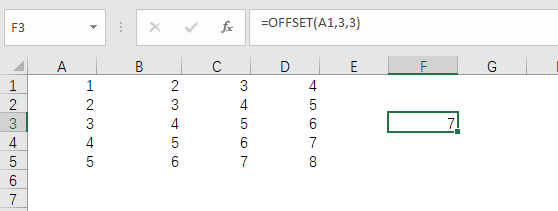=OFFSET(参照值，MATCH(),MATCH())

• 第一个MATCH(),用来确定偏移的行数

• 第二个MATCH(),用来确定偏移的列数

=OFFSET(181,MATCH(J183,182:193,0),MATCH(K183,181:181,0))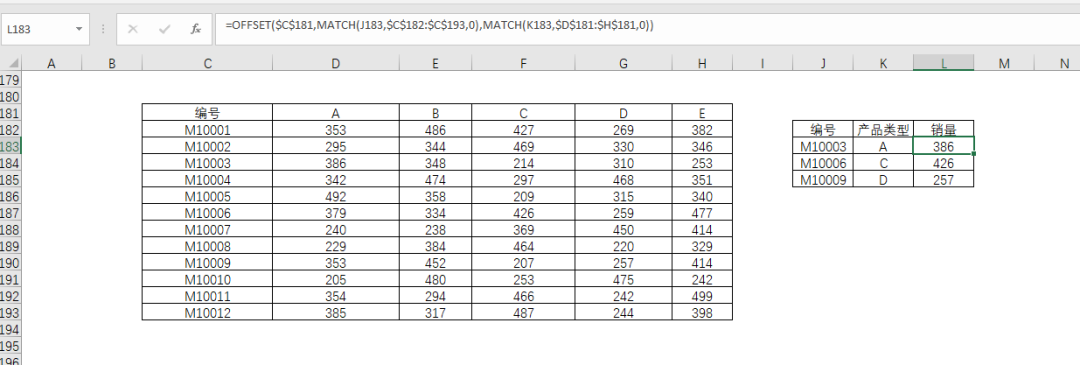### 4.2 MATCH与VLOOKUP函数组合

=VLOOKUP(I2,1:11,MATCH(J2,1:1,0),0)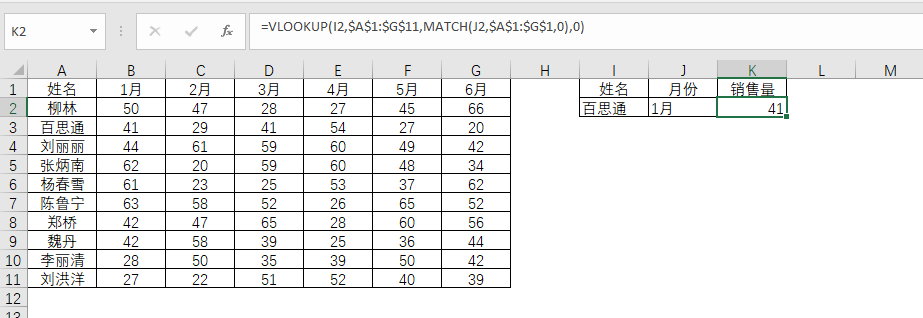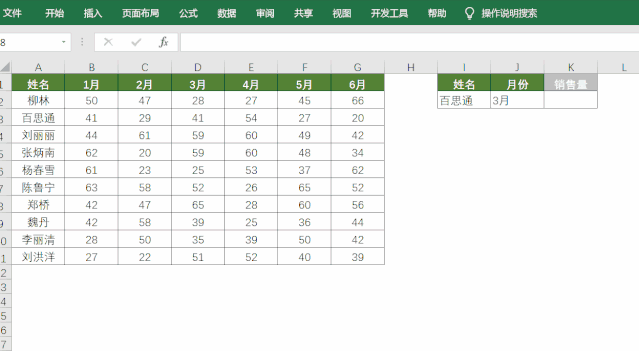### 4.3 MATCH与INDEX函数组合

INDEX函数用于在一个区域中，根据指定的行和列号来返回内容。

=INDEX(单元格区域，指定的行数，指定的列数)

=INDEX(11:15,3,4)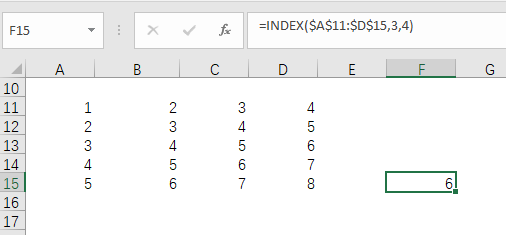4.3.1 正向查询

=INDEX(24:33,MATCH(D24,24:33,0))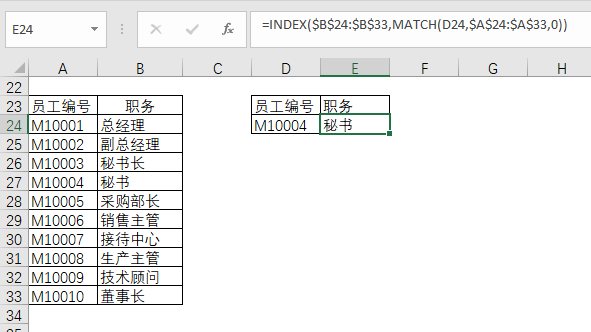4.3.2 逆向查询

=INDEX(39:48,MATCH(D39,39:48,0))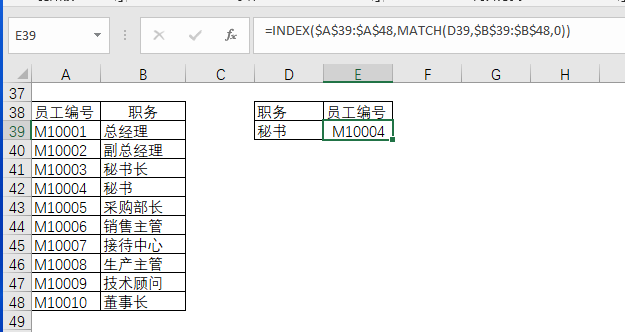4.3.3 多条件查询

=INDEX(39:48,MATCH(F55&G55,55:64&55:64,0))